Shubham Kumar ShuklaShubham9455

8min

# Common Smoothing Filters

Here we will learn about different channels utilized for picture smoothing, which is fundamental for getting great precision. When a picture is clear we get better data and information from it. Our machine resembles our mind. Like our cerebrum, we train our machine relative to some information. When we get clear information we can adapt effectively. In bygone times we utilized highly contrasting TV that did not perceive subtleties plainly, however, now we have shaded and LED TV, and the pixels are clear and smooth. Additionally, in the past we used pictures straightforwardly for gathering data yet the precision of legitimate data was bad, but now there are numerous algorithms like averaging, Gaussian filters, and etc. described below.

### Image smoothing

Image smoothing is useful for increasing accuracy of our machine learning models.

In the code implementation below you can see we are importing a predefined library of open-cv and Python for converting image data to binary value data in matrix form. The shape of the matrix depends on how high quality you wish to make the image and information.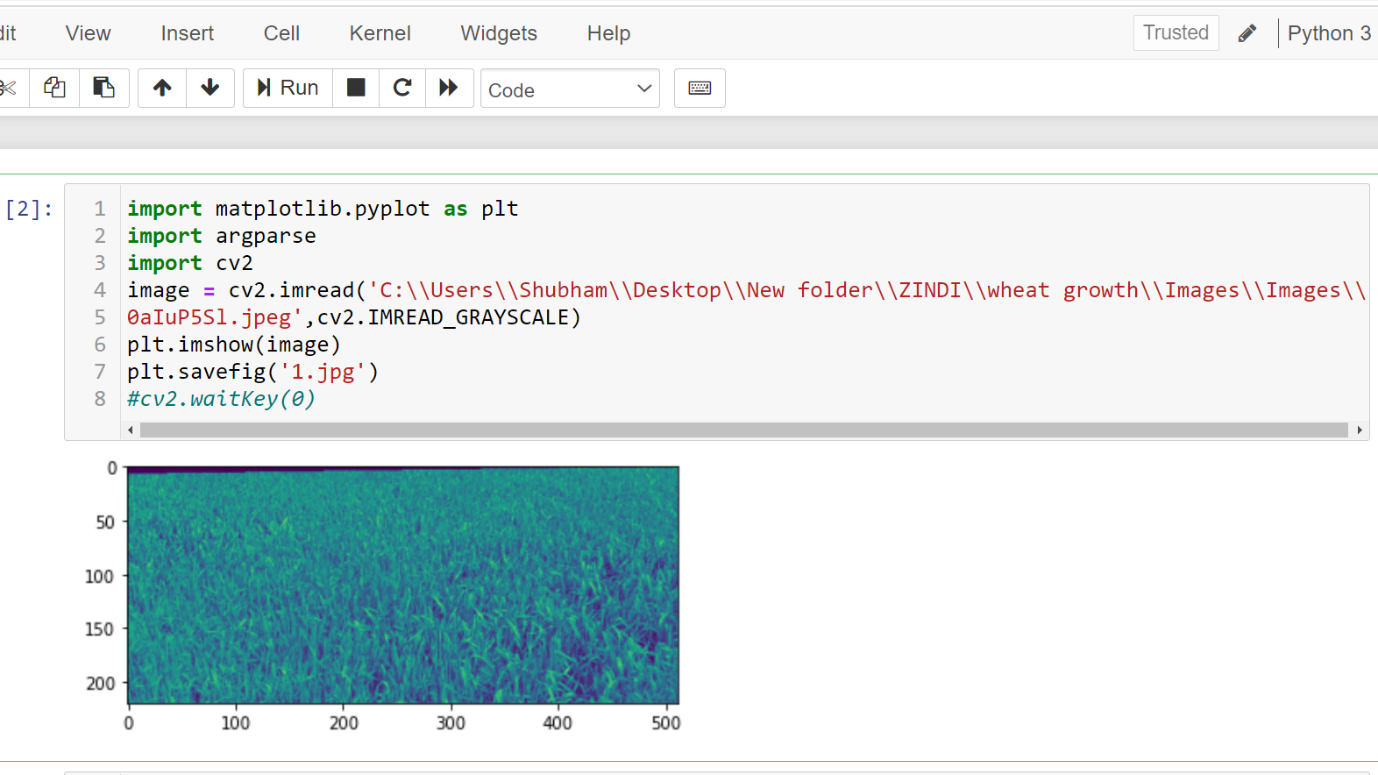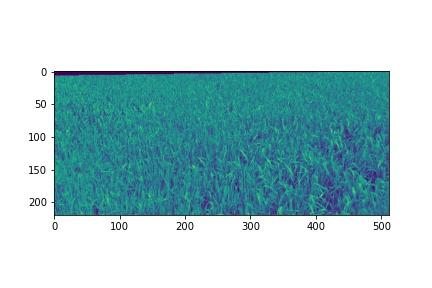In the above image you will see that when we plot it there is some information that is not clear, so we use various filters to clear and smooth the image.

### AVERAGING:

Averaging filter is simple in that we take basically the mean of neighboring pixel, this pixel is used for removing noise, it basically blurs our image. In matrix binary converted data how will we know there is noise? The answer is very simple - when any value in the matrix is negative that negative value is noise. There are many types of noise including salt-pepper noise, etc.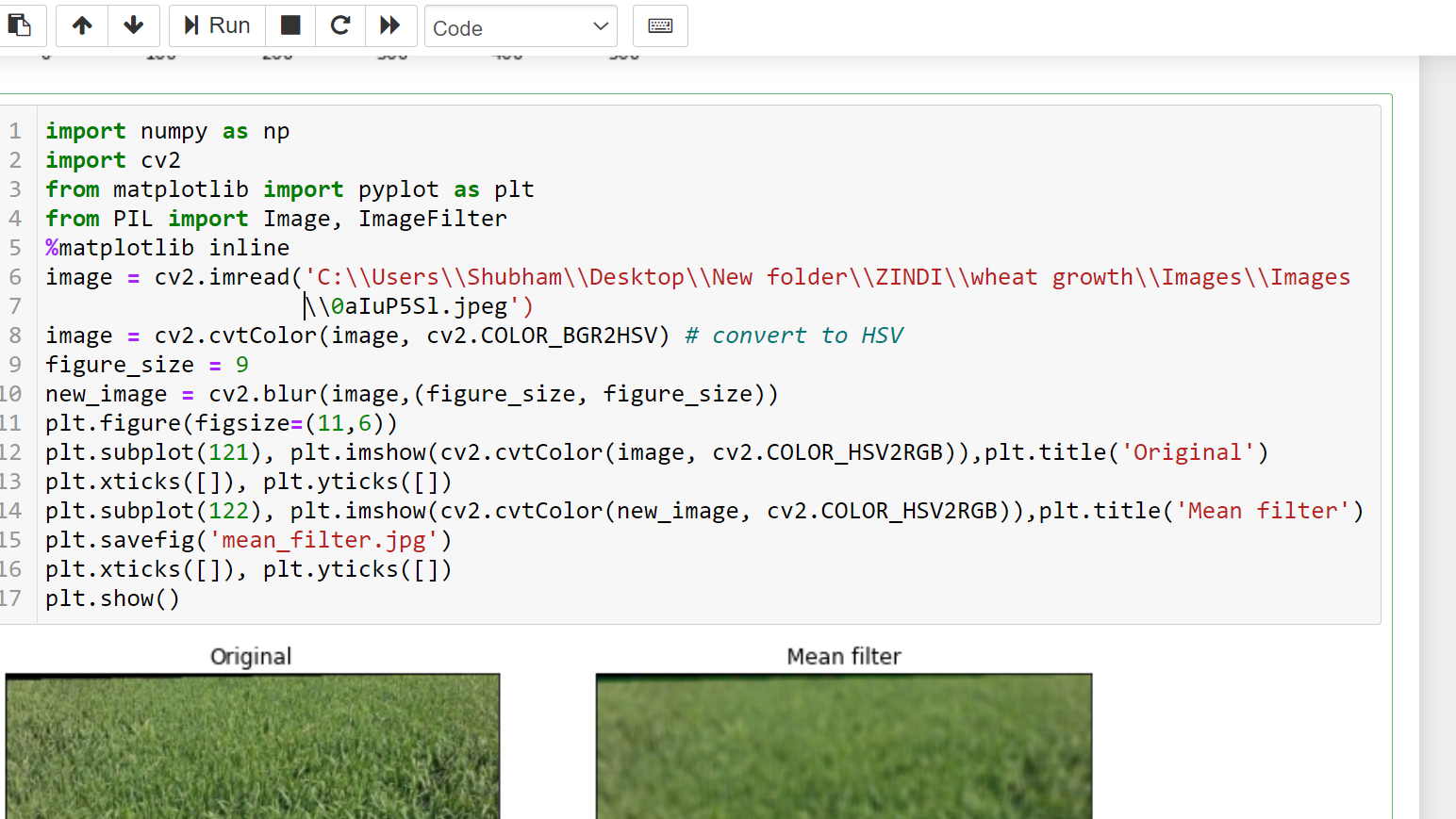#### Smoothing channels:

Gaussian:

Like the mean filter, Gaussian filter also takes the average of the pixels but there is a proper function which applies on each pixel. Below is the function used. Like the mean filter it is also used for noise removal and blurs the image. But, it stores the information of the image clearly while it blurs the edge of the pixel.

• The loads are tests of a 2D Gaussian capacity: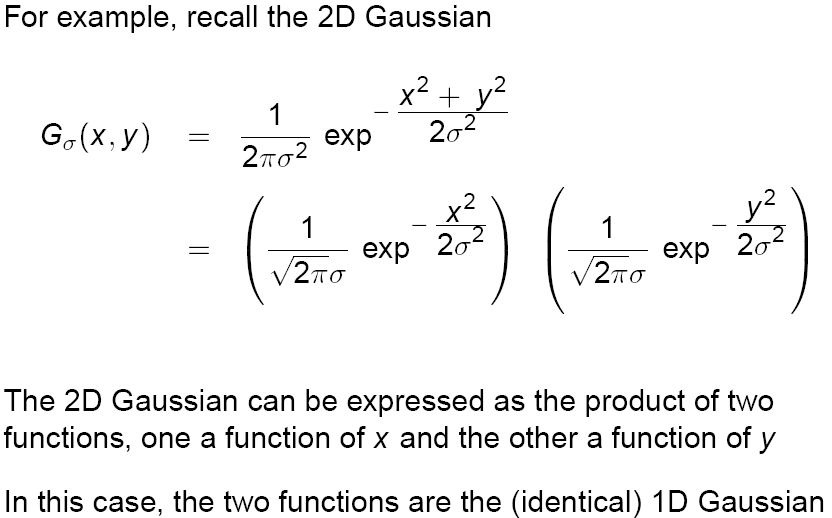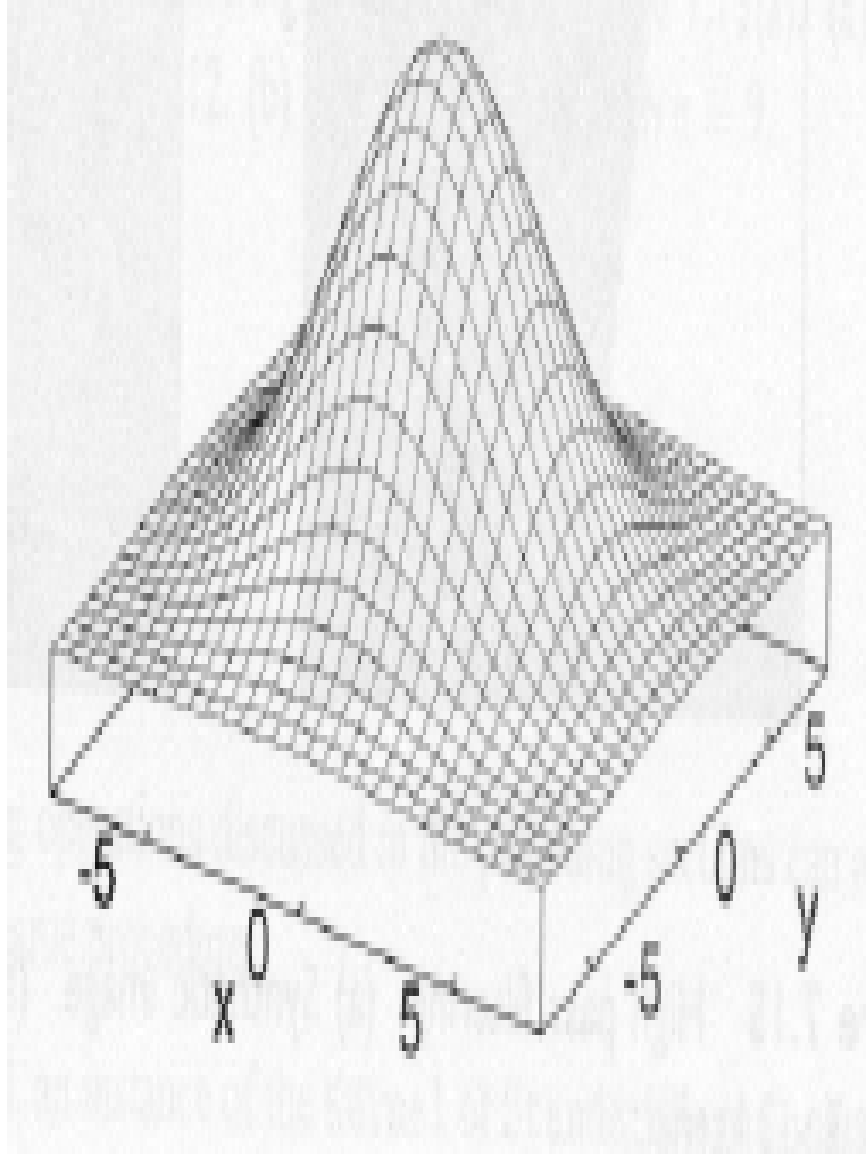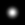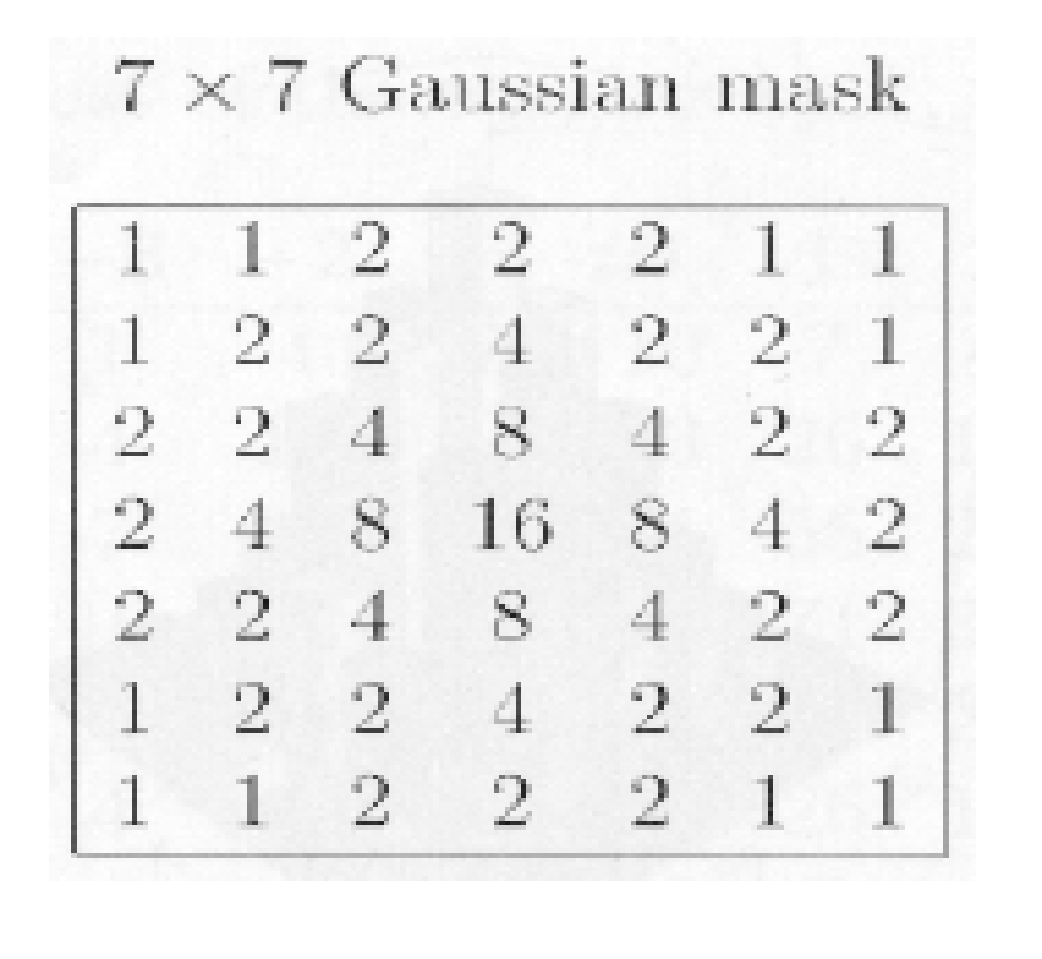• σ  controls the measures of smoothing

• σ = 1.4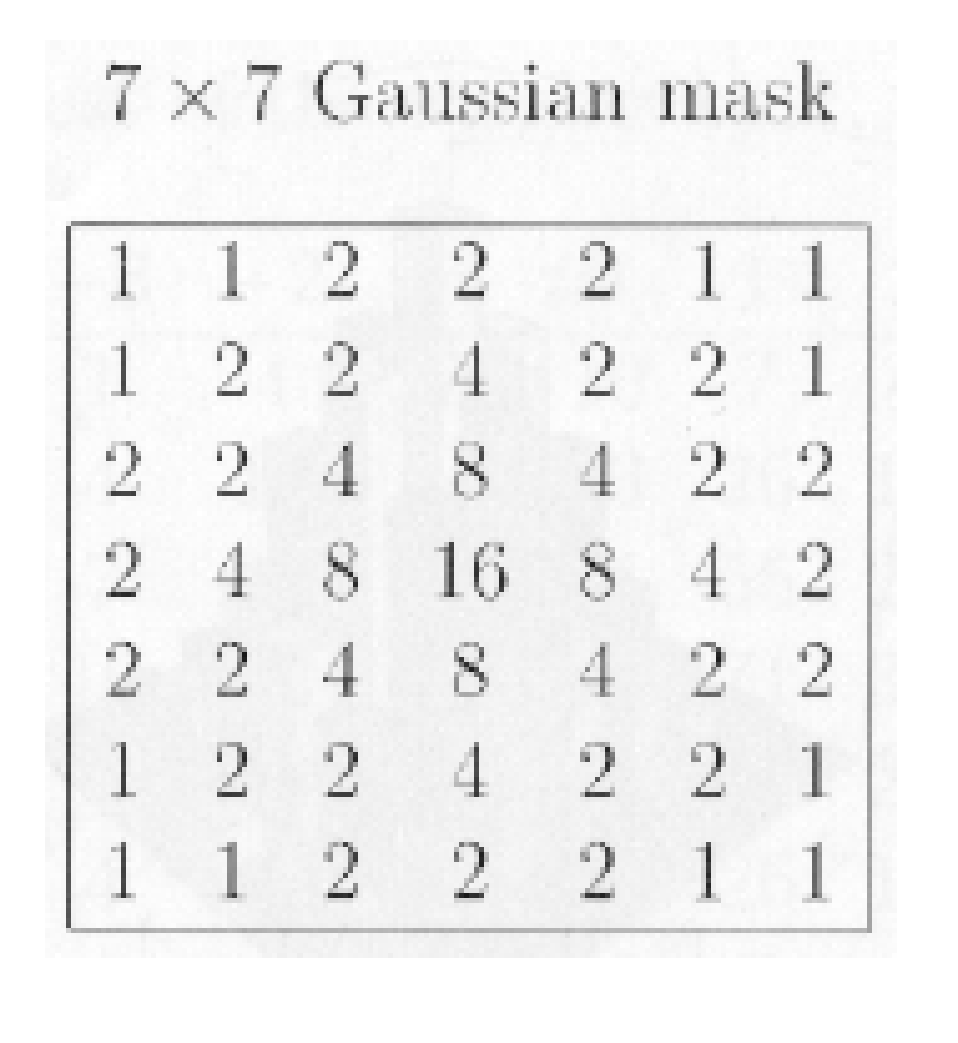• σ = 3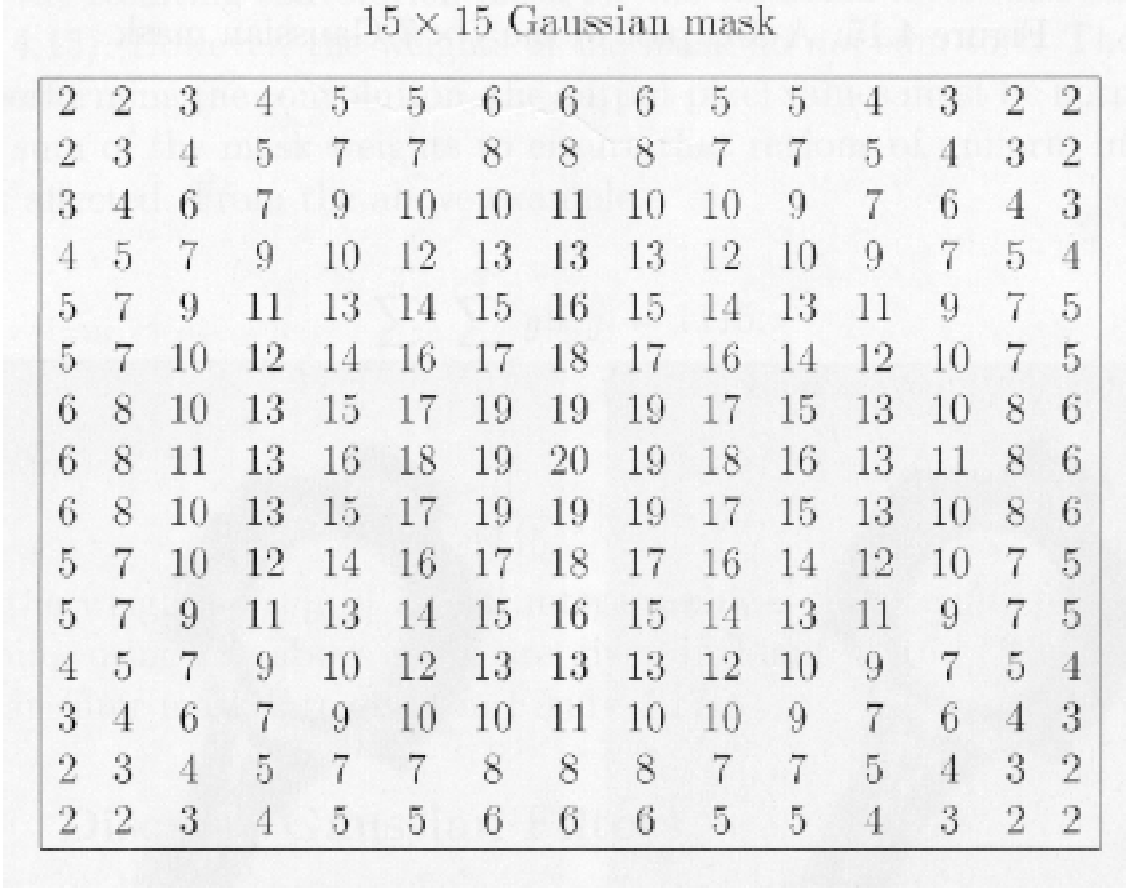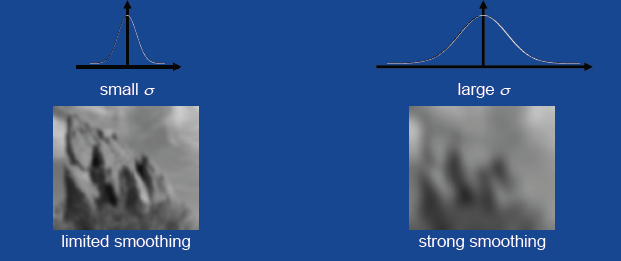#### Averaging versus Gaussian Smoothing:

Here we plainly see what I mentioned above - that the Gaussian filter is better than a mean filter because it stores the information of the image more clearly.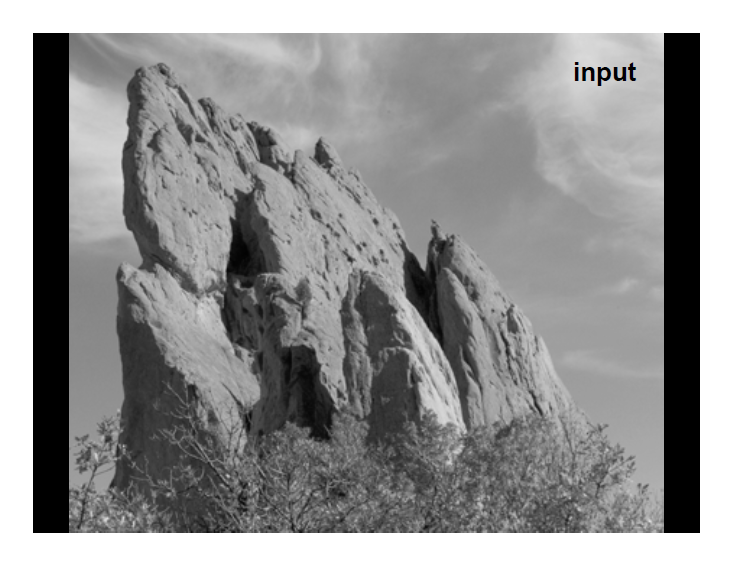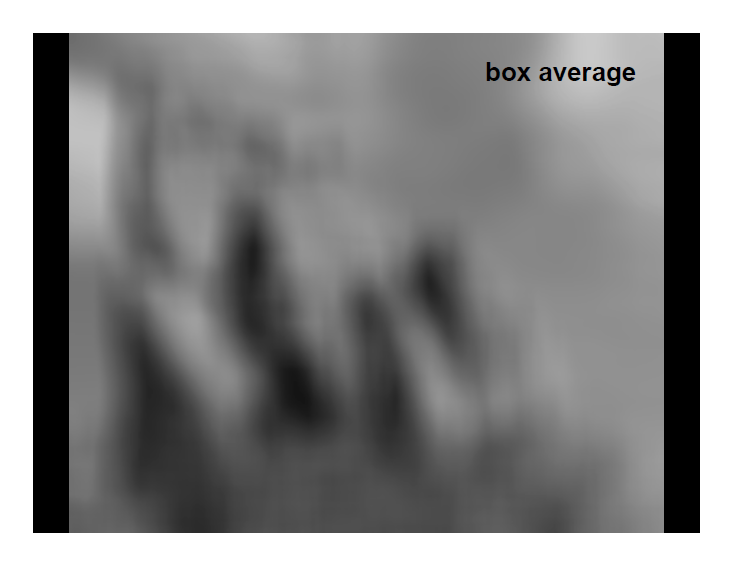Averaging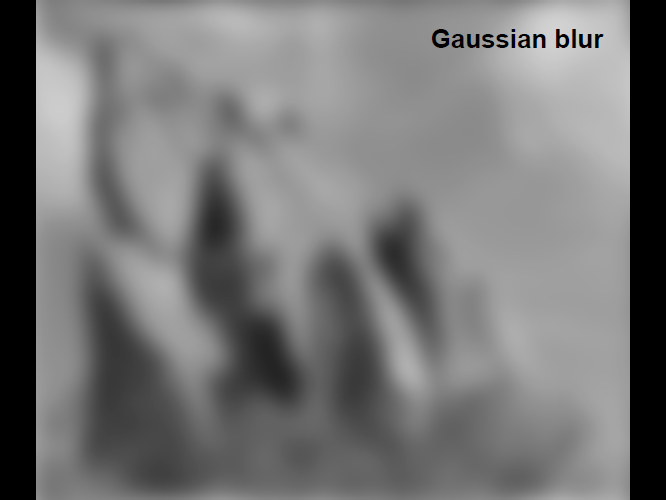Gaussian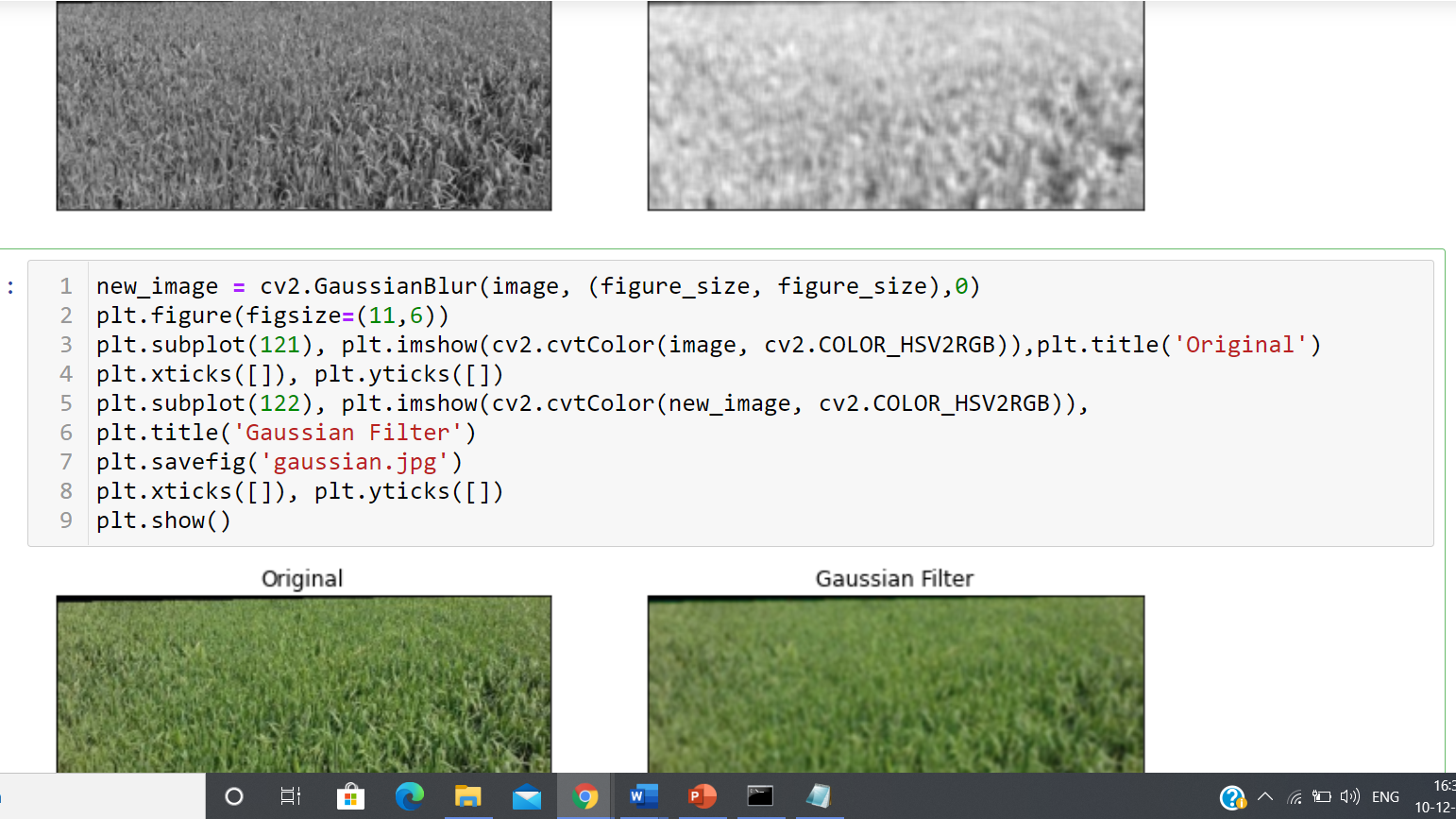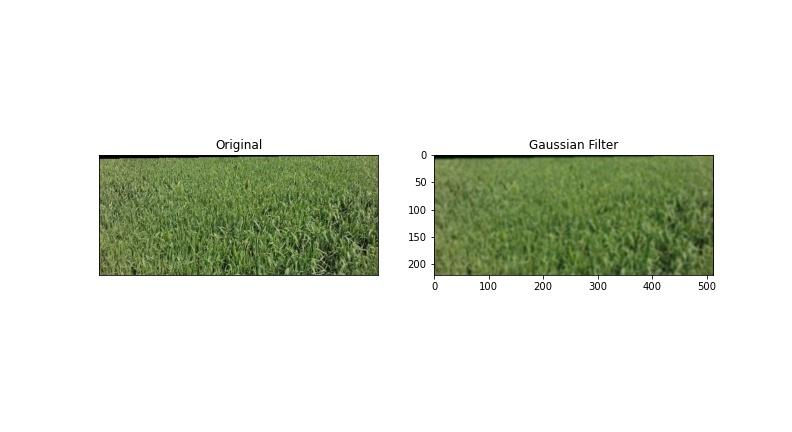#### Smoothing Filters: Median Filtering

**(non-straight):

Like the mean filter, the median filter takes the median value of neighboring pixels which are around our central pixel. Median filter is better than both mean and Gaussian filters.

It is very successful at eliminating salt and pepper commotion (i.e., arbitrary events of high contrast pixels).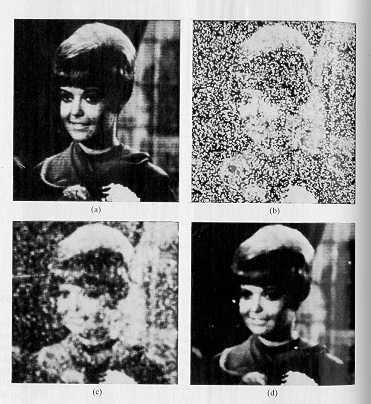Averaging                                     middle channel• Replace every pixel by the middle in a neighborhood around the pixel.

• The size of the near pixels controls the measures  of smoothing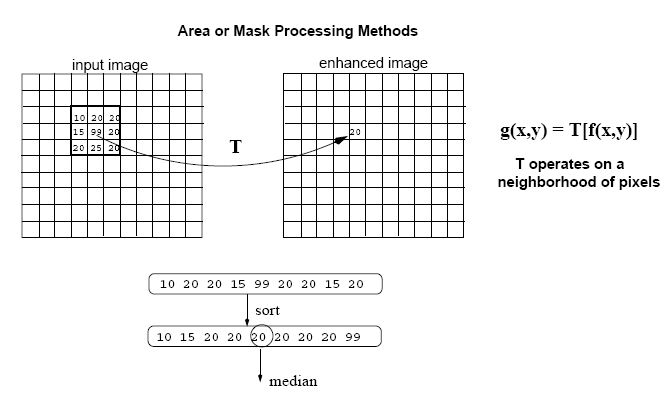• Sharpening Filters (high-pass):
Helpful for featuring fine subtleties.

• The components of the cover contain both positive and negative loads.

• Mask components entirety  to 0.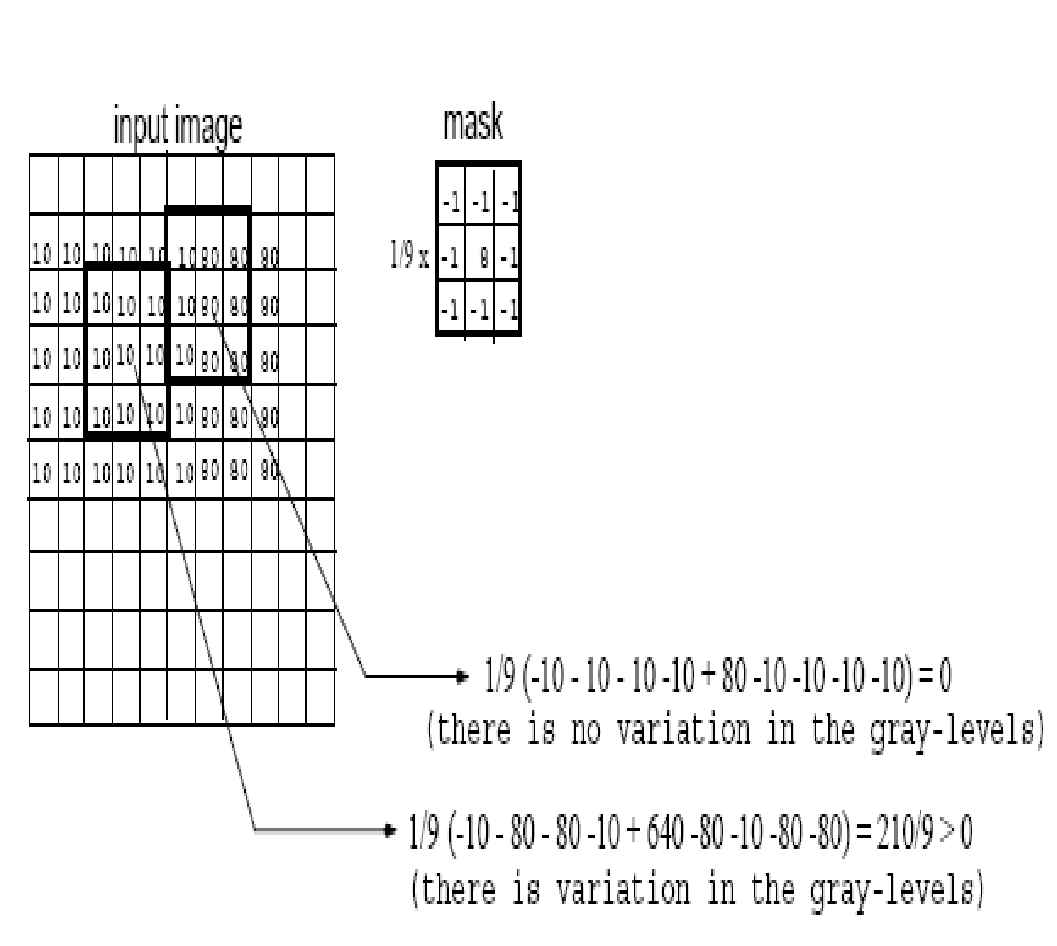Honing Filters – Example:

Here we used a honing filter in the place of a smoothing filter. Because of plagiarism it detects:

info picture                        hone picture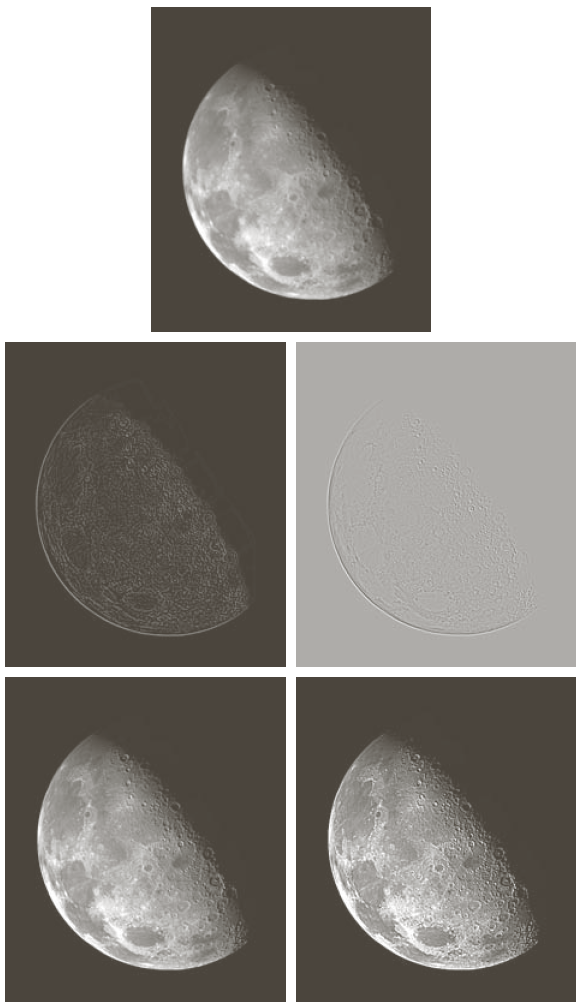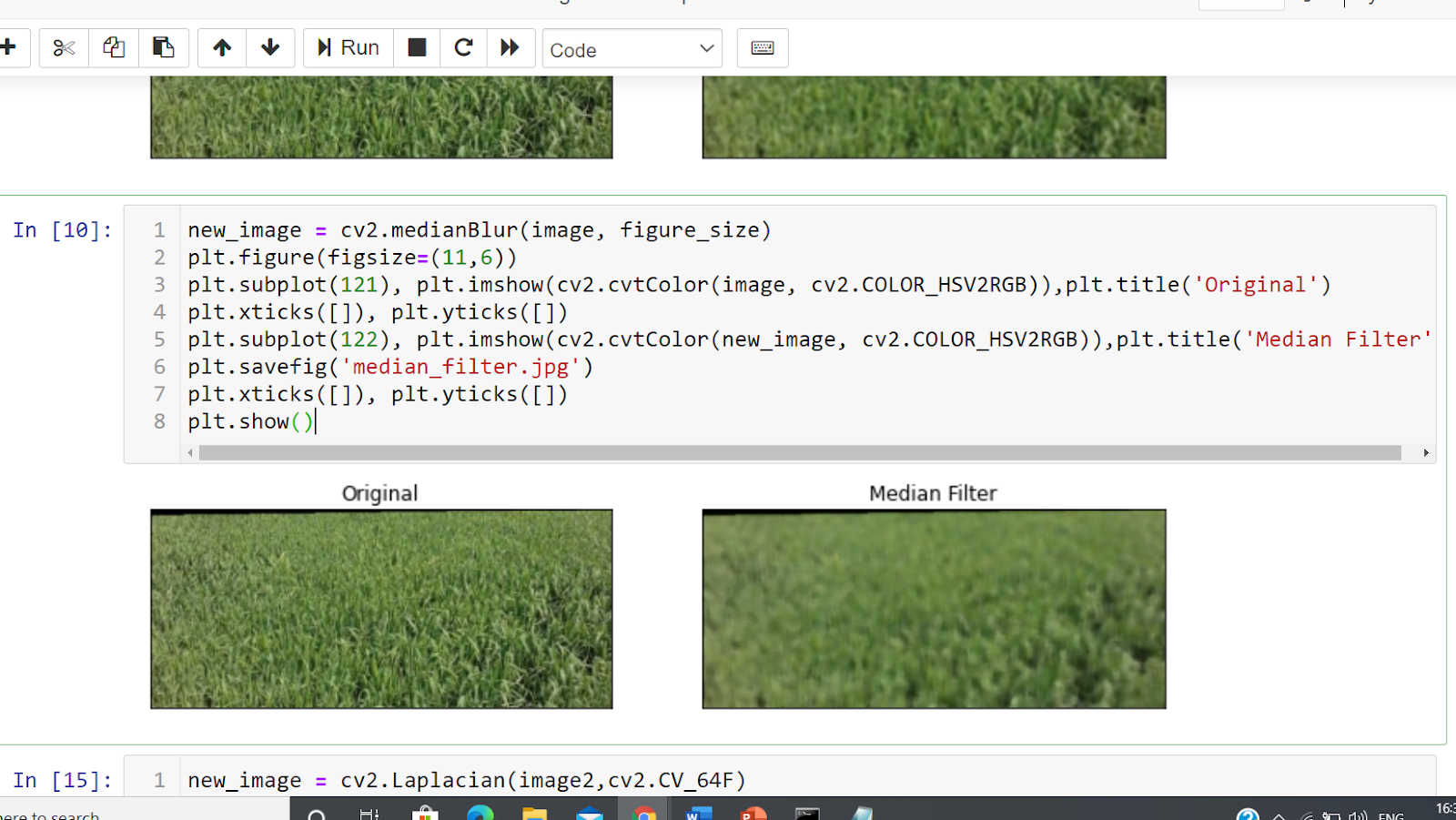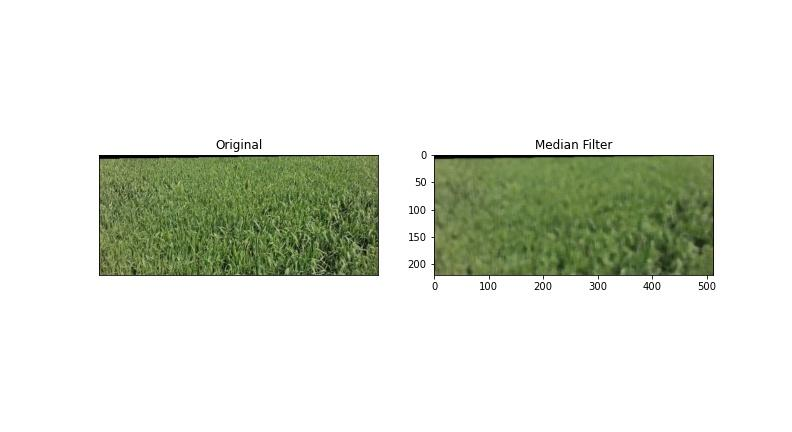Common Sharpening Filters:

• Unsharp concealing

• High Boost channel

• Gradient (first subsidiary)

• Laplacian (second subordinate)

**Honing Filters: Unsharp Masking  **

• Obtain a sharp picture by taking away a lowpass sifted (i.e., smoothed) picture from the first picture: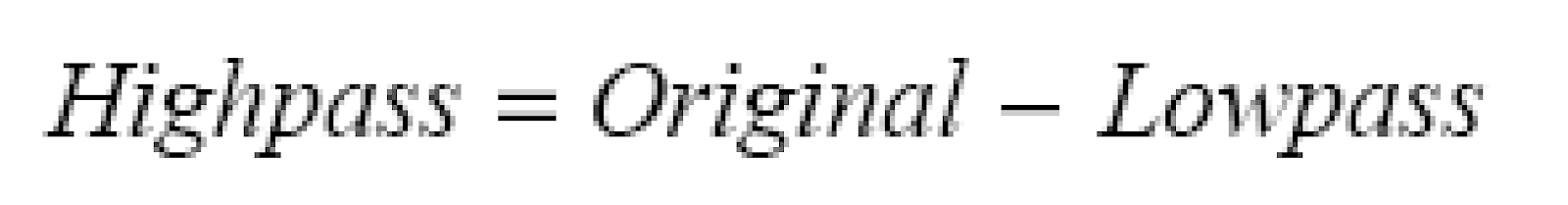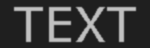_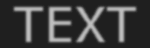=****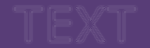With high differentiation upgrade.

**Honing Filters: High Boost  **

If A=1, the outcome is unsharp covering.

• If A>1, some portion of the first picture is added back to the high pass sifted HIGH BOOST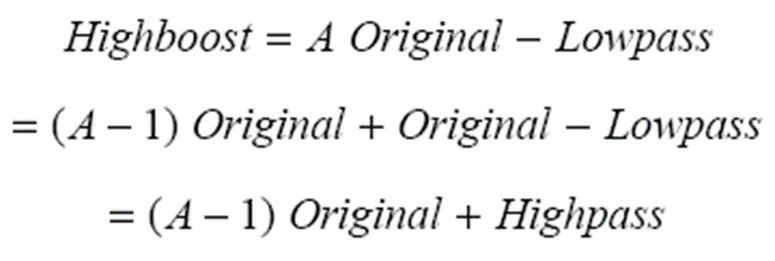.

One approach to actualize support sifting is utilizing veil underneath: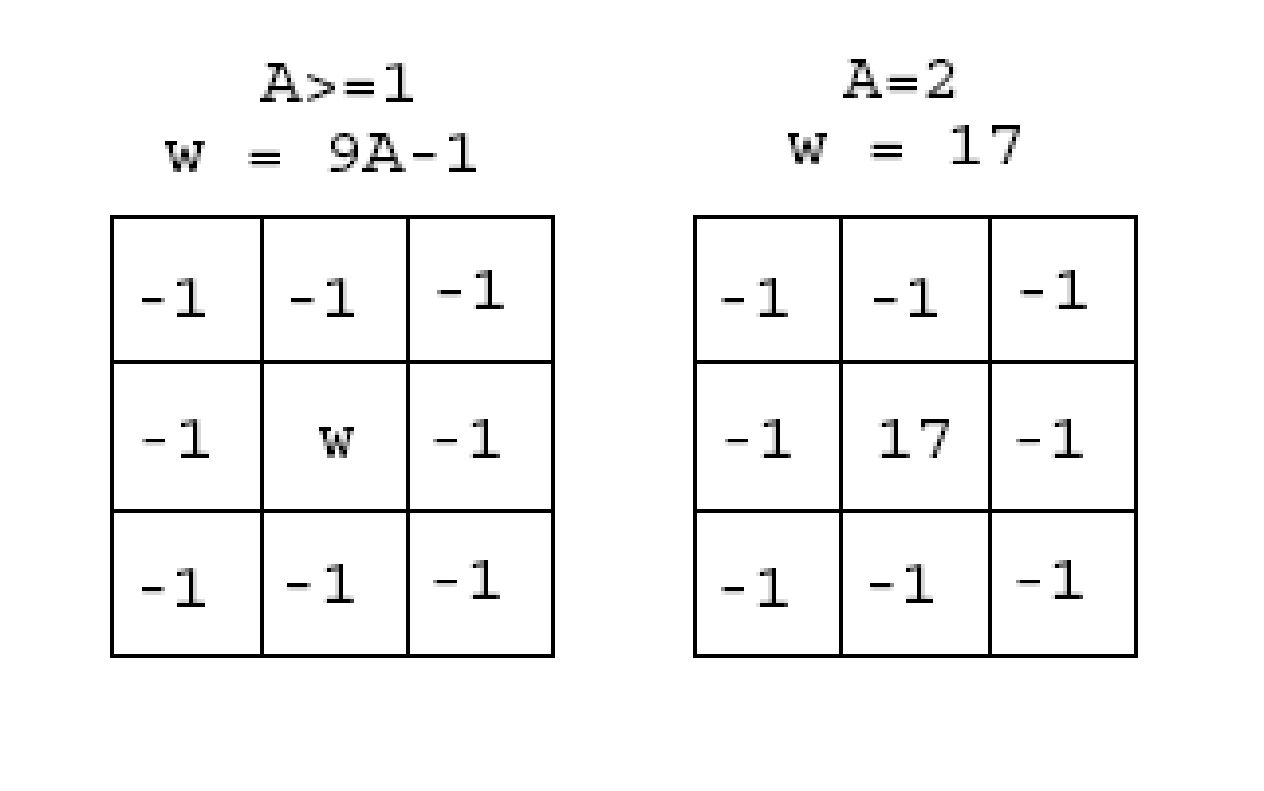Honing Filters: Derivatives

• The derivative of a picture brings about a smooth image.

• Image derivatives can be calculated using the slope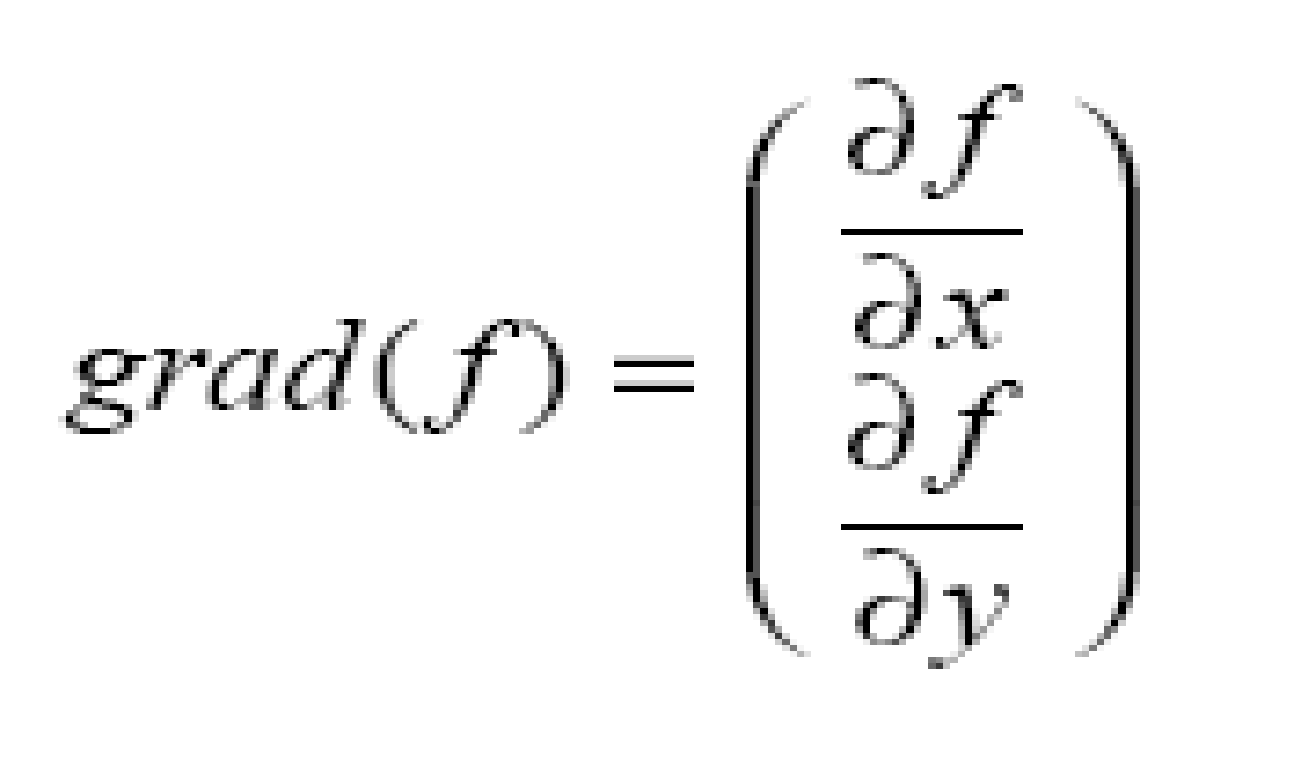Slope:

• The slope is a vector which has size and direction: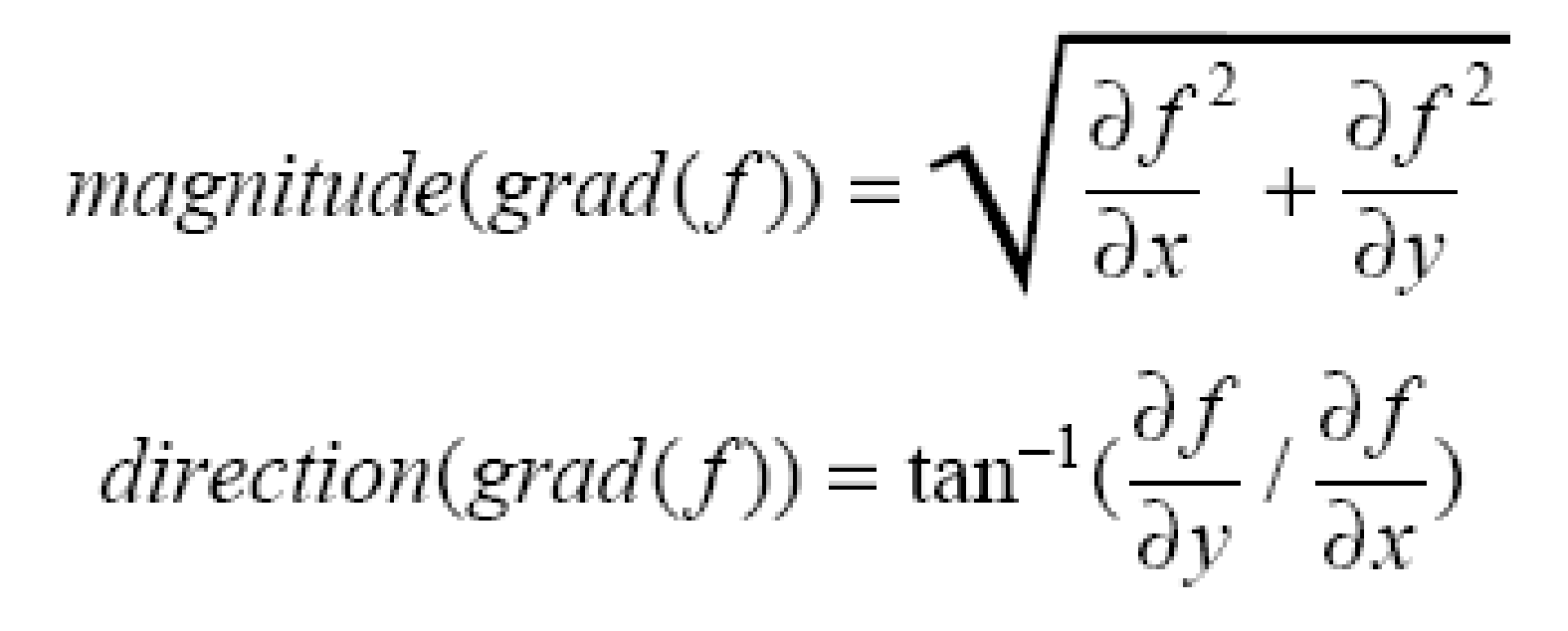• Slope value: gives data about edge strength.

• Slope vector: make 90 degrees to the vector of the edge.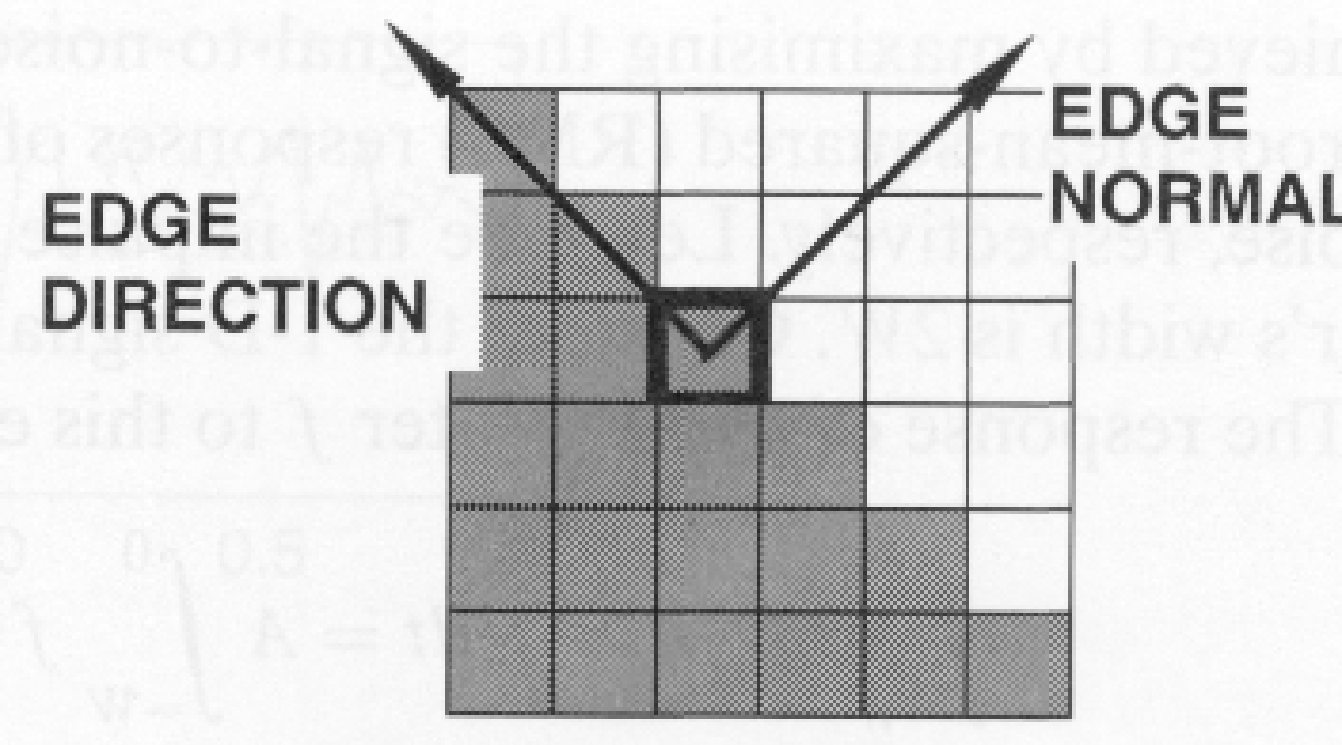Below is the code implementation of edge detection. With the help of that we can easily detect the edge of our image because all the information of the image is in the edge and central pixels.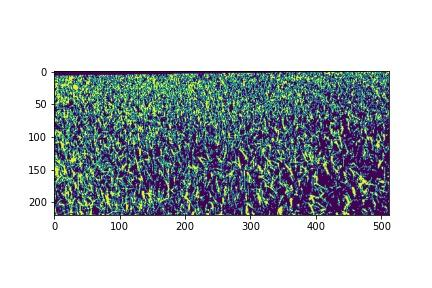Slope calculation:

• Approximate incomplete subordinates utilizing limited contrasts: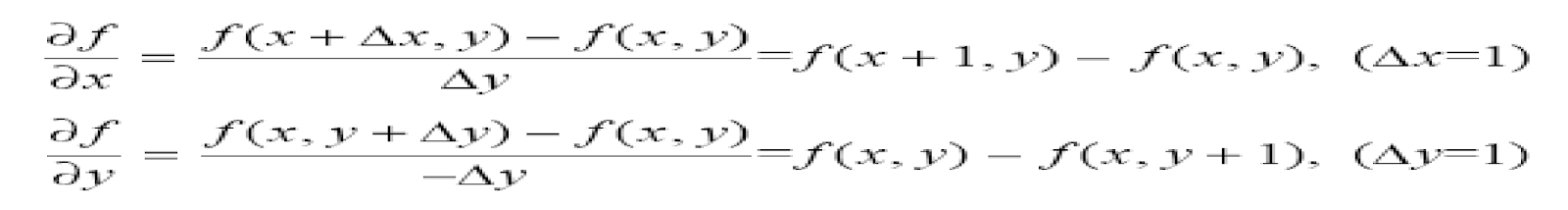Eg: look below the fractional subsidiaries: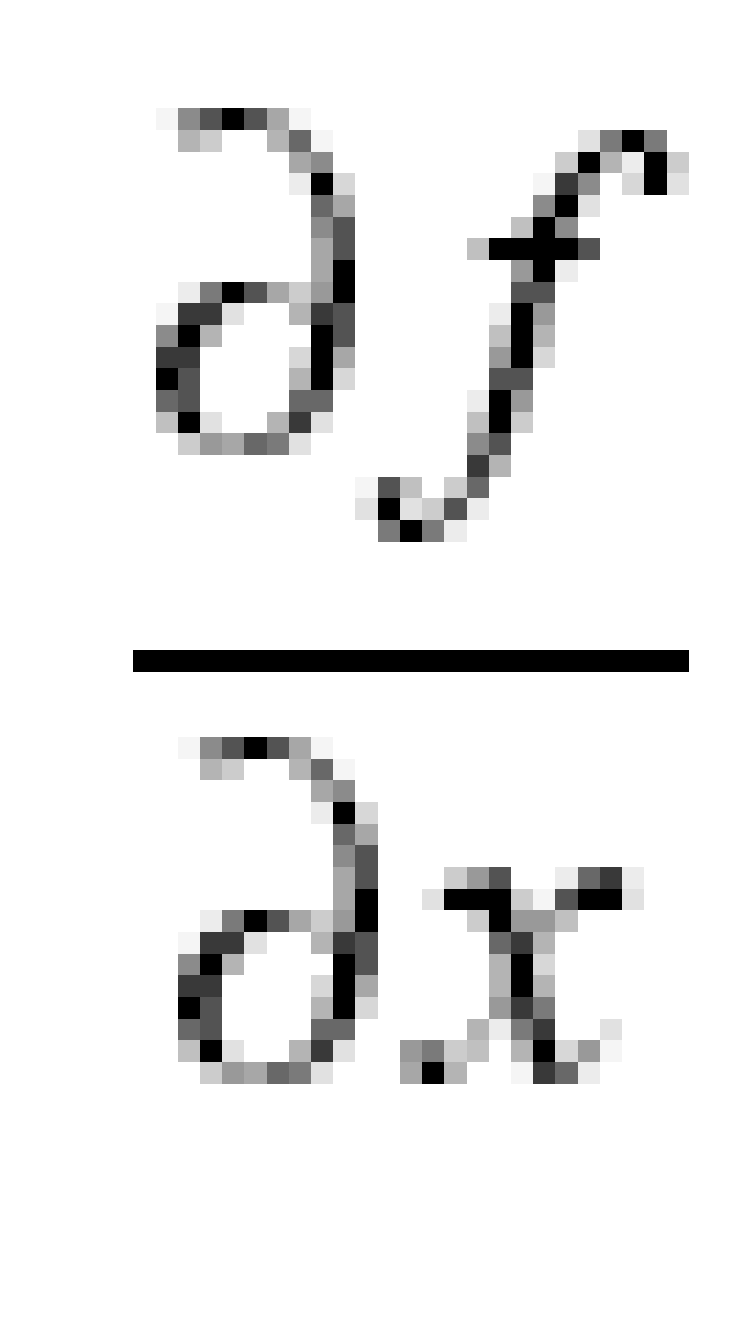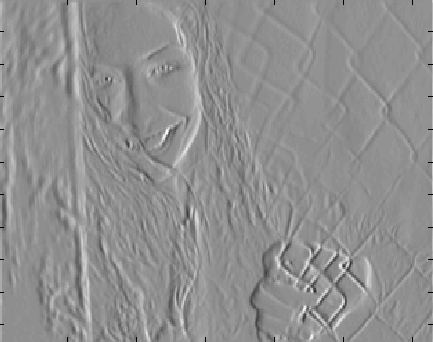horizontal edge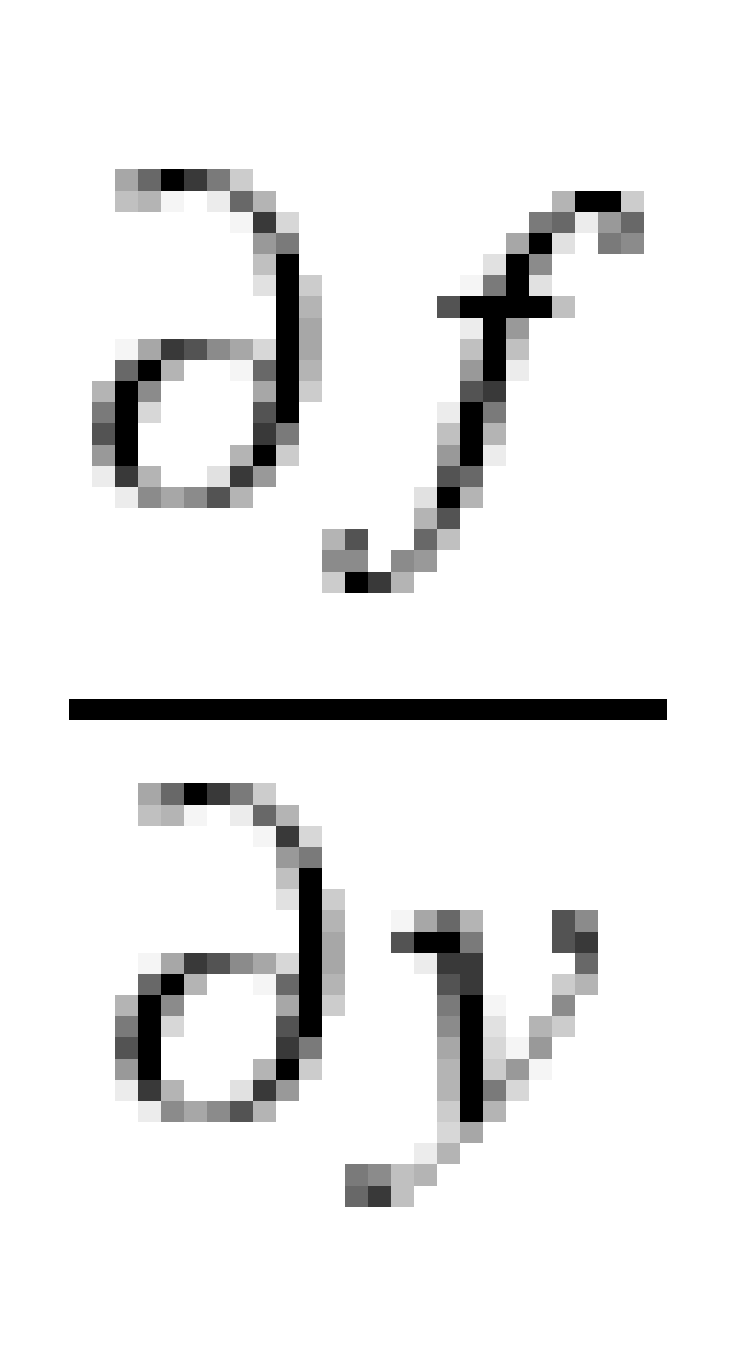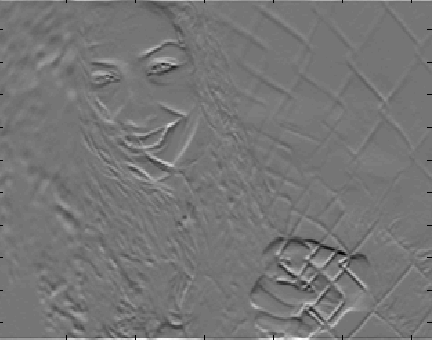vertical edgeAn alternate estimation of the inclination

Above we use two functions. One is differential with respect to x which is used for horizontal edge detection, the other is with respect to y for vertical edge detection.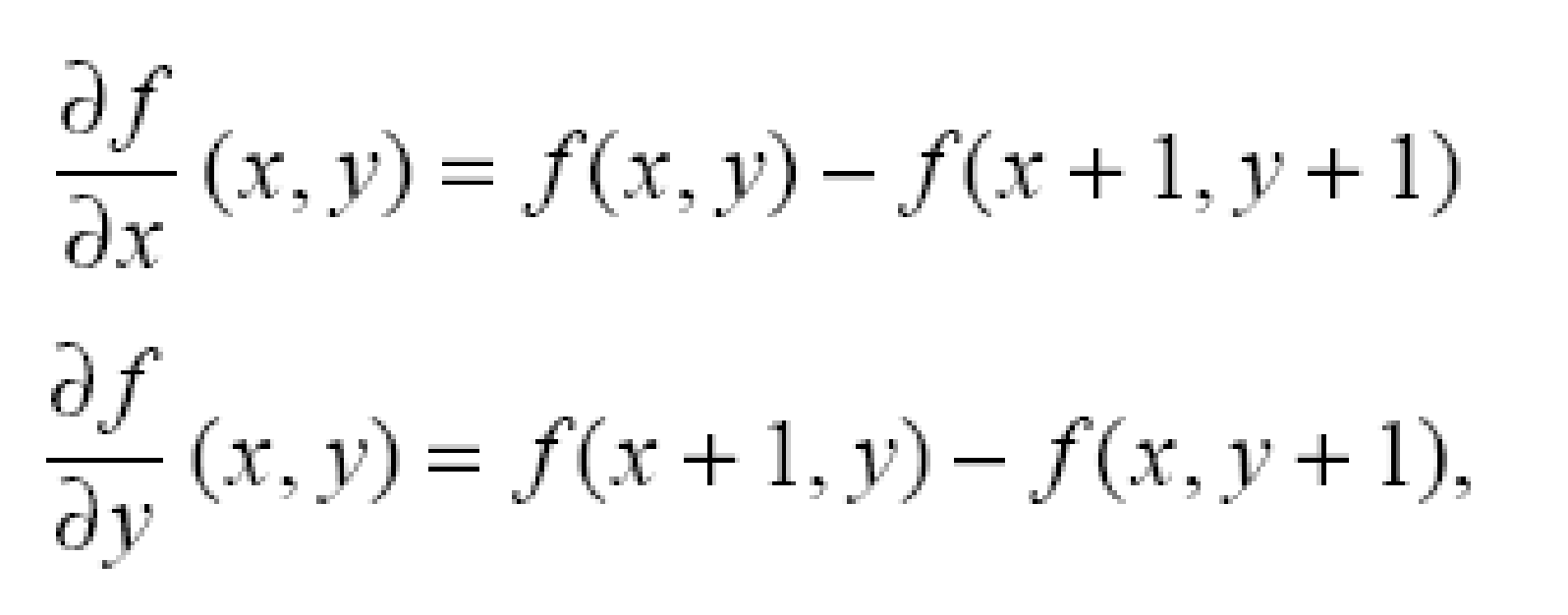Great approx.• We can execute df/dx and df/dy below: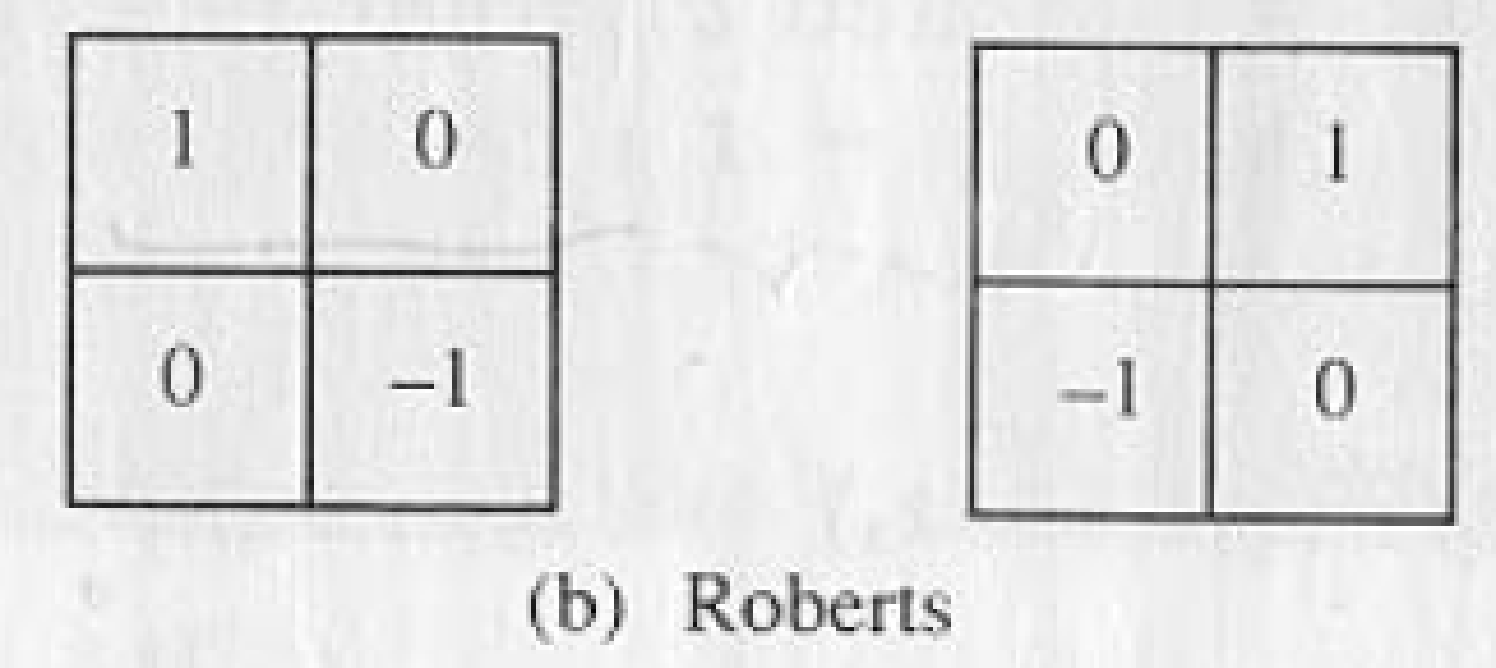Execute  Gradient Using Masks :

• Other approximations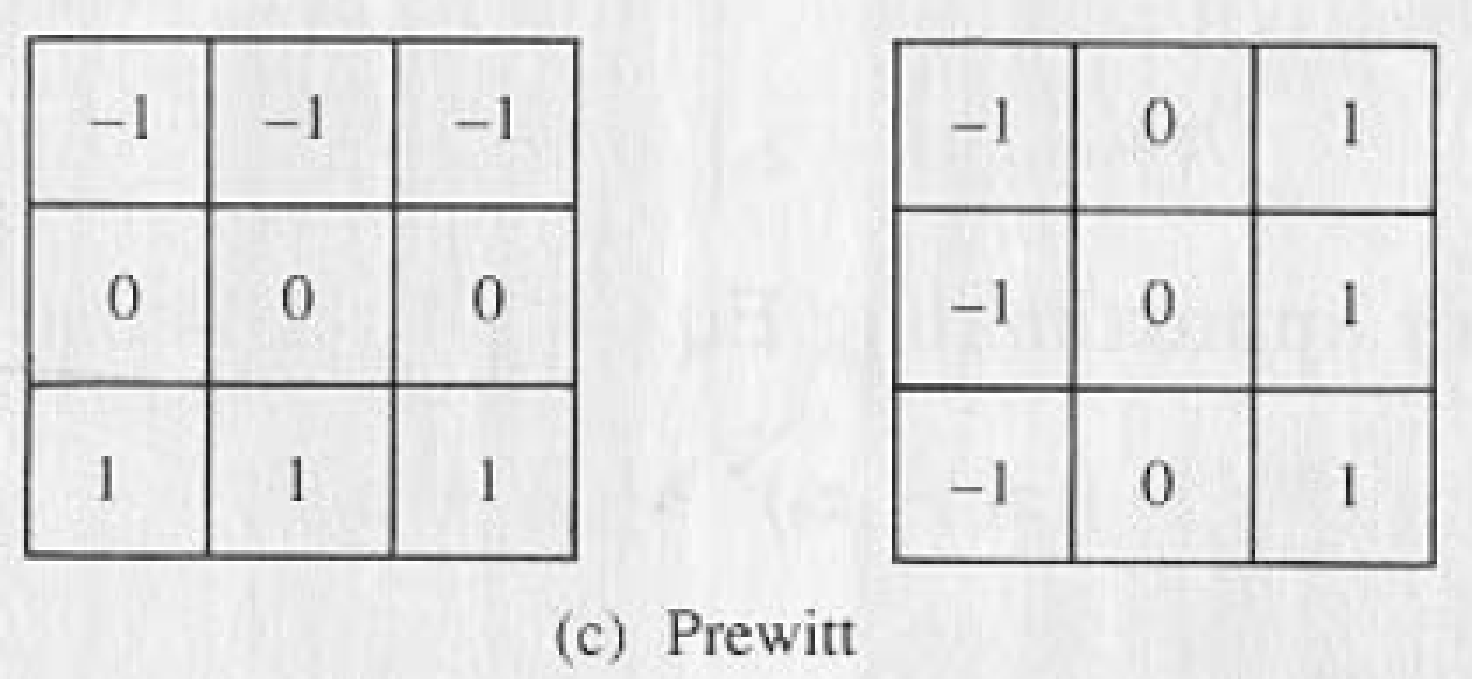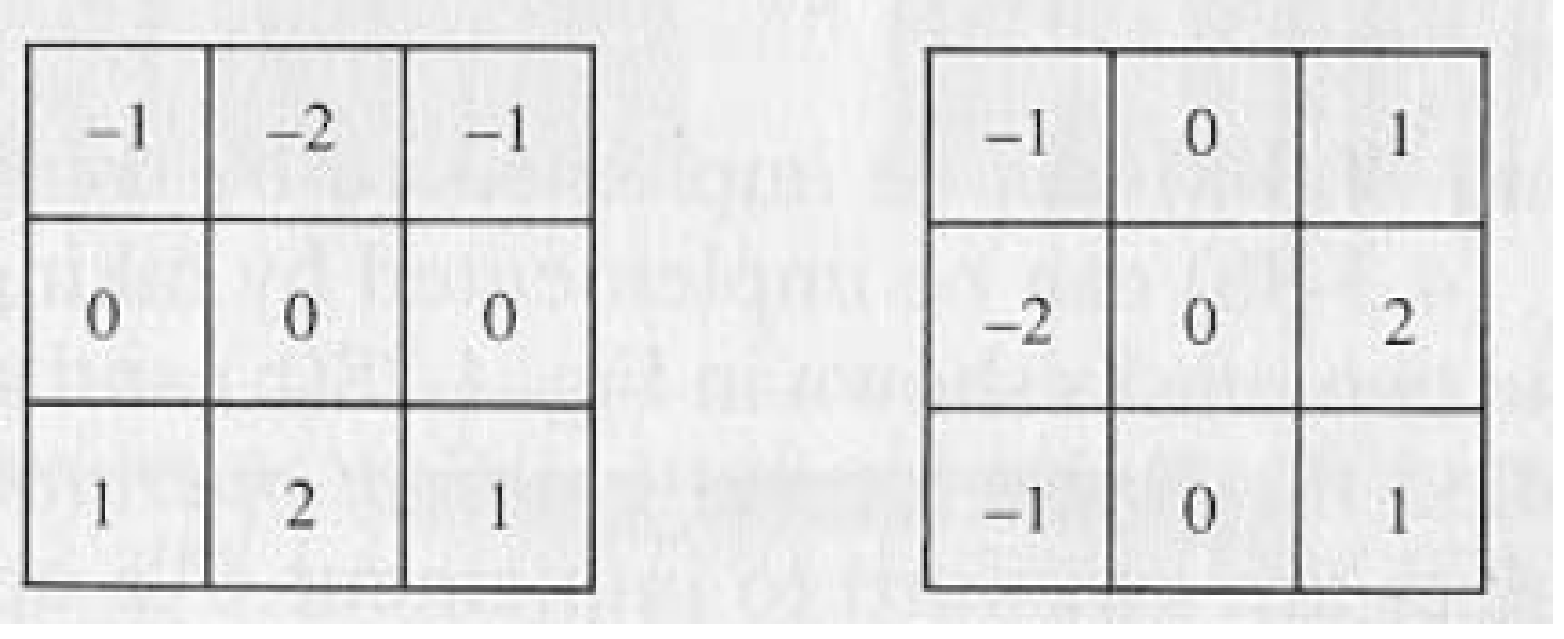sobel

Laplacian:

This filter is useful when there is a sudden change in intensities. Then this filter is useful for edge detection.

The Laplacian (second subsidiary) is characterized as: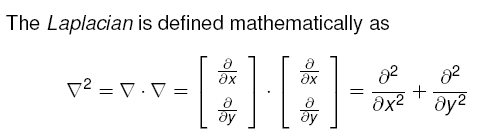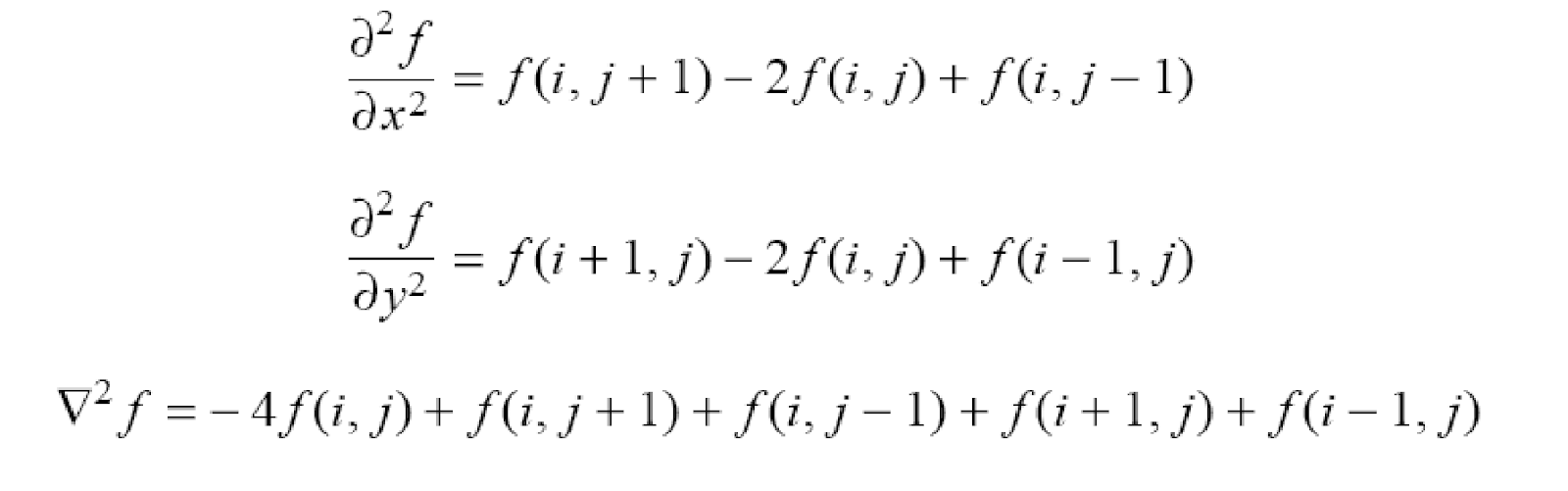Laplacian (cont’d)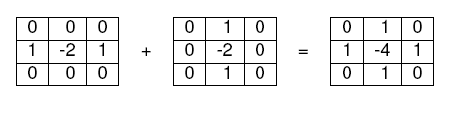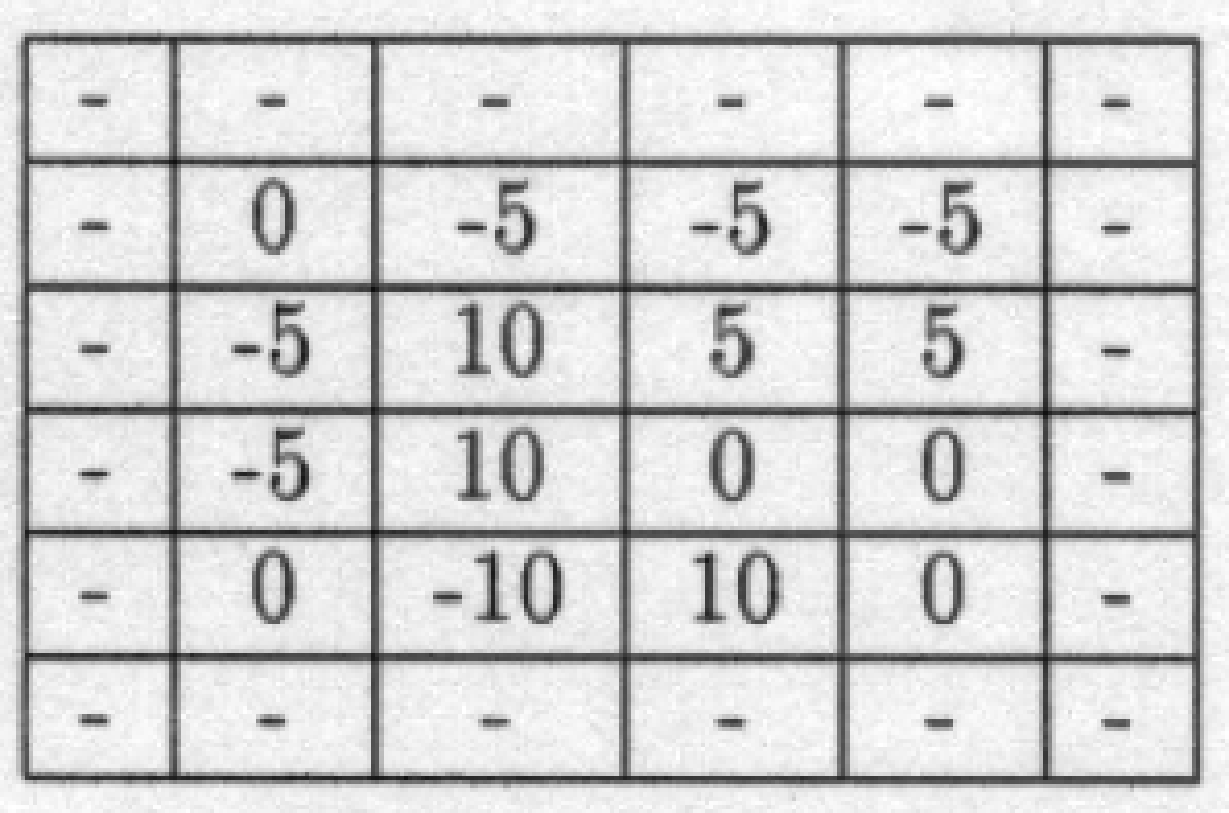Edges can be found by identifying the zero-intersections.

Below is the code implementation of the Laplacian filter, how it is used, and the current quality of the image after applying that filter.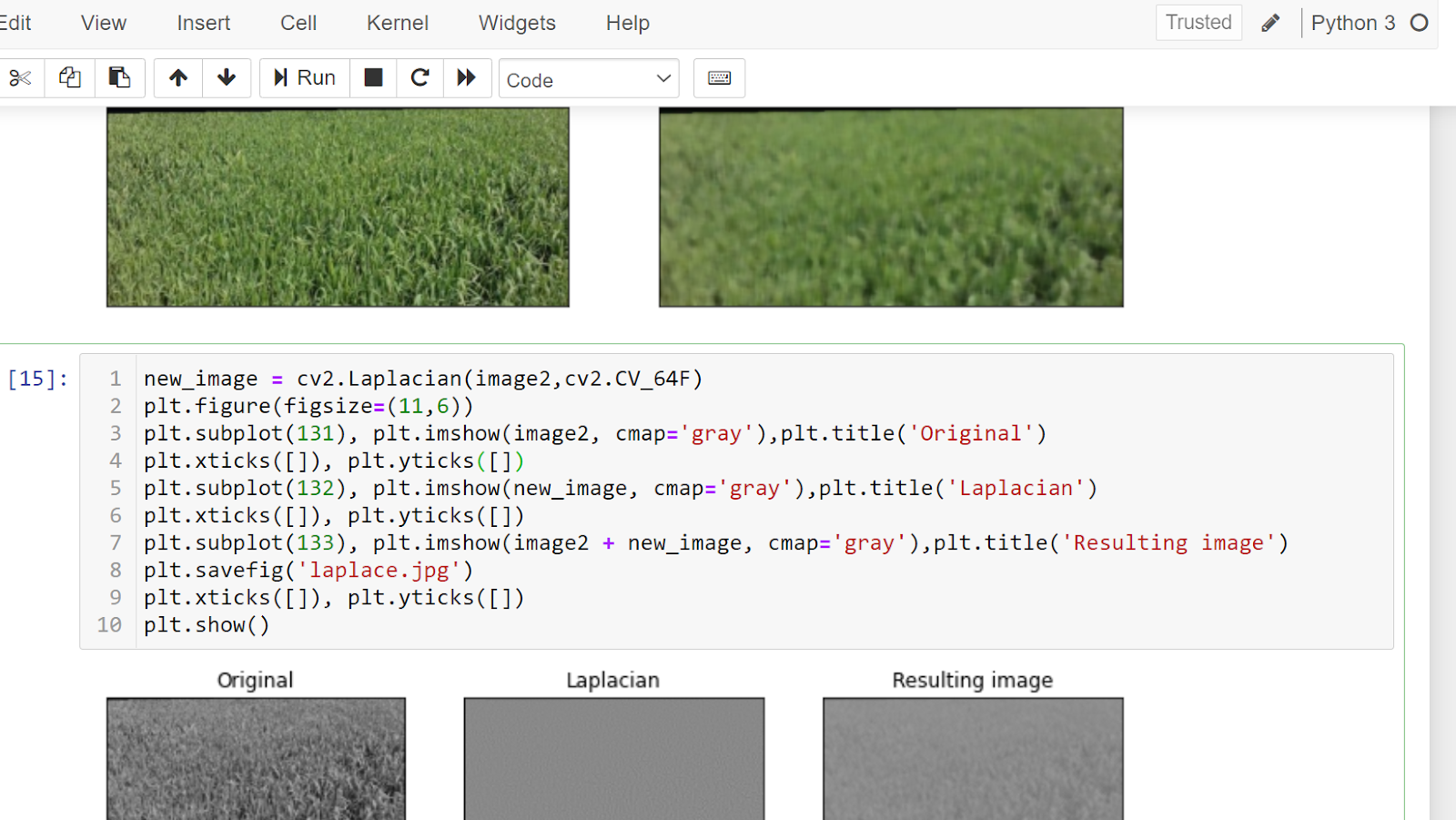Thank you for reading. The above is very general theory and basic maths implementation so anyone can easily understand it.